Students can download 11th Business Maths Chapter 1 Matrices and Determinants Ex 1.2 Questions and Answers, Notes, Samcheer Kalvi 11th Business Maths Guide Pdf helps you to revise the complete Tamilnadu State Board New Syllabus, helps students complete homework assignments and to score high marks in board exams.

## Tamilnadu Samacheer Kalvi 11th Business Maths Solutions Chapter 1 Matrices and Determinants Ex 1.2

### Samacheer Kalvi 11th Business Maths Matrices and Determinants Ex 1.2 Text Book Back Questions and Answers

Question 1.
Find the adjoint of the matrix A = $$\left[\begin{array}{ll} 2 & 3 \\ 1 & 4 \end{array}\right]$$
Solution:
A = $$\left[\begin{array}{ll} 2 & 3 \\ 1 & 4 \end{array}\right]$$
Adj A = $$\left[\begin{array}{rr} 4 & -3 \\ -1 & 2 \end{array}\right]$$

Question 2.
If A = $$\left[\begin{array}{lll} 1 & 3 & 3 \\ 1 & 4 & 3 \\ 1 & 3 & 4 \end{array}\right]$$ then verify that A(adj A) = |A| I and also find A-1.
Solution:
Given A = $$\left[\begin{array}{lll} 1 & 3 & 3 \\ 1 & 4 & 3 \\ 1 & 3 & 4 \end{array}\right]$$
|A| = $$\left|\begin{array}{lll} 1 & 3 & 3 \\ 1 & 4 & 3 \\ 1 & 3 & 4 \end{array}\right|$$
= $$1\left|\begin{array}{ll} 4 & 3 \\ 3 & 4 \end{array}\right|-3\left|\begin{array}{ll} 1 & 3 \\ 1 & 4 \end{array}\right|+3\left|\begin{array}{ll} 1 & 4 \\ 1 & 3 \end{array}\right|$$
= 1[16 – 9] – 3[4 – 3] + 3[3 – 4]
= 1(7) – 3(1) + 3(-1)
= 7 – 3 – 3
= 1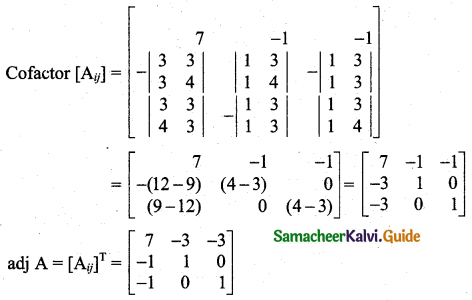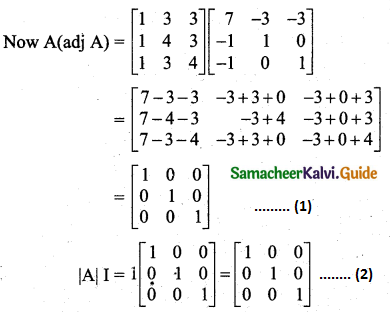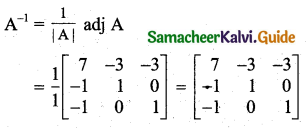From (1) and (2), A(Adj A) = |A| IQuestion 3.
Find the inverse of each of the following matrices:
(i) $$\left[\begin{array}{rr} 1 & -1 \\ 2 & 3 \end{array}\right]$$
(ii) $$\left[\begin{array}{rr} 3 & 1 \\ -1 & 3 \end{array}\right]$$
(iii) $$\left[\begin{array}{lll} 1 & 2 & 3 \\ 0 & 2 & 4 \\ 0 & 0 & 5 \end{array}\right]$$
(iv) $$\left[\begin{array}{rrr} -3 & -5 & 4 \\ -2 & 3 & -1 \\ 1 & -4 & -6 \end{array}\right]$$
Solution:
(i) $$\left[\begin{array}{rr} 1 & -1 \\ 2 & 3 \end{array}\right]$$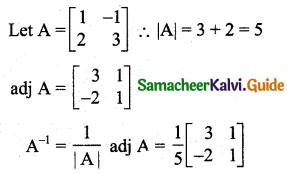(ii) $$\left[\begin{array}{rr} 3 & 1 \\ -1 & 3 \end{array}\right]$$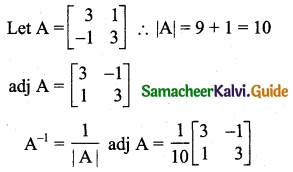(iii) $$\left[\begin{array}{lll} 1 & 2 & 3 \\ 0 & 2 & 4 \\ 0 & 0 & 5 \end{array}\right]$$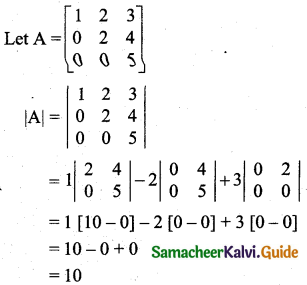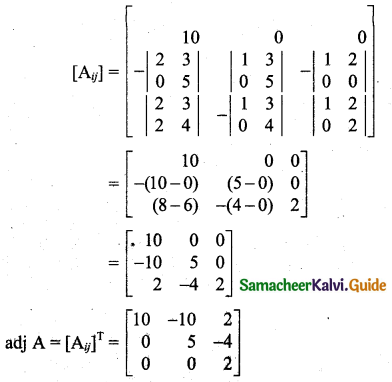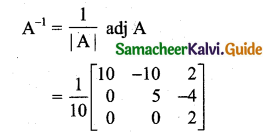(iv) $$\left[\begin{array}{rrr} -3 & -5 & 4 \\ -2 & 3 & -1 \\ 1 & -4 & -6 \end{array}\right]$$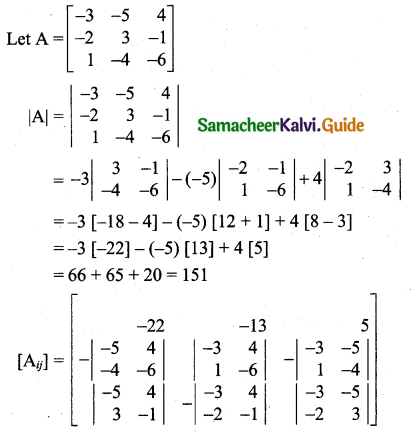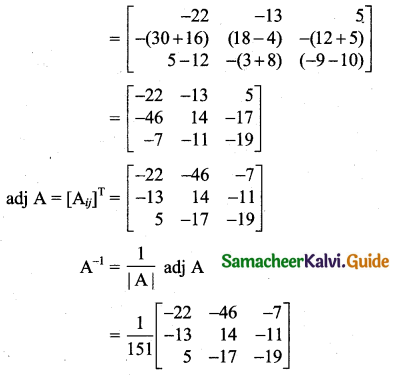Question 4.
If A = $$\left[\begin{array}{rr} 2 & 3 \\ 1 & -6 \end{array}\right]$$ and B = $$\left[\begin{array}{rr} -1 & 4 \\ 1 & -2 \end{array}\right]$$, then verify adj(AB) = (adj B) (adj A).
Solution: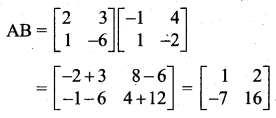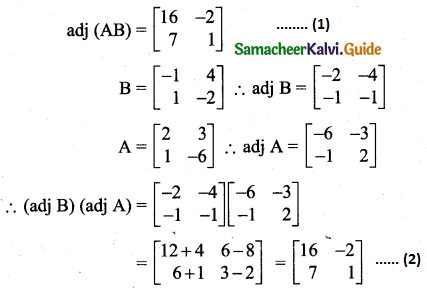Question 5.
If A = $$\left[\begin{array}{rrr} 2 & -2 & 2 \\ 2 & 3 & 0 \\ 9 & 1 & 5 \end{array}\right]$$ then, show that (adj A) A = O.
Solution: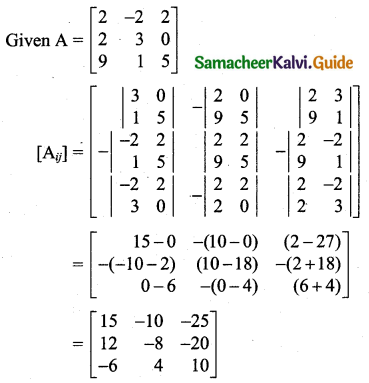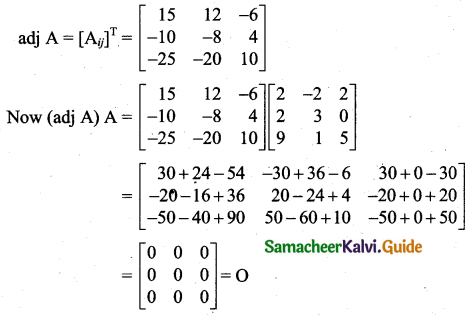Question 6.
If A = $$\left[\begin{array}{rrr} -1 & 2 & -2 \\ 4 & -3 & 4 \\ 4 & -4 & 5 \end{array}\right]$$ then, show that the inverse of A is A itself.
Solution: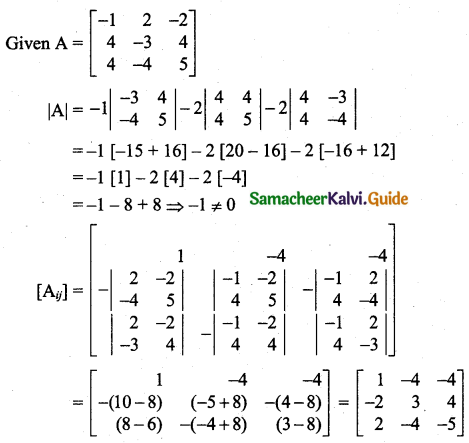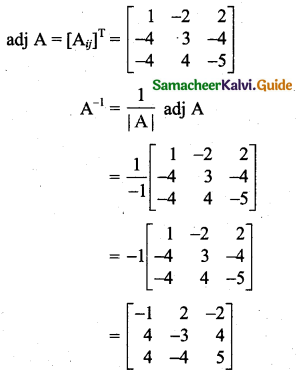∴ A-1 = A
Hence proved.Question 7.
If A-1 = $$\left[\begin{array}{rrr} 1 & 0 & 3 \\ 2 & 1 & -1 \\ 1 & -1 & 1 \end{array}\right]$$ then, find A.
Solution:
Given A-1 = $$\left[\begin{array}{rrr} 1 & 0 & 3 \\ 2 & 1 & -1 \\ 1 & -1 & 1 \end{array}\right]$$
We know that (A-1)-1 = A
So we have to find inverse of A-1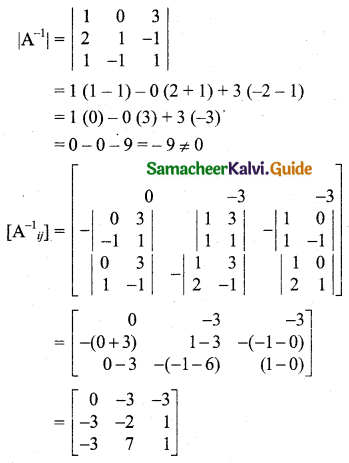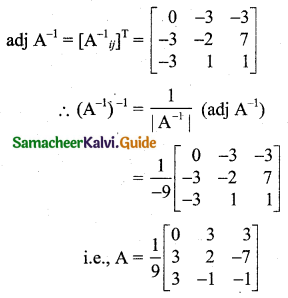Question 8.
Show that the matrices A = $$\left[\begin{array}{lll} 2 & 2 & 1 \\ 1 & 3 & 1 \\ 1 & 2 & 2 \end{array}\right]$$ and B = $$\left[\begin{array}{ccc} \frac{4}{5} & \frac{-2}{5} & \frac{-1}{5} \\ \frac{-1}{5} & \frac{3}{5} & \frac{-1}{5} \\ \frac{-1}{5} & \frac{-2}{5} & \frac{4}{5} \end{array}\right]$$ are inverses of each other.
Solution:
To prove that A and B are inverses of each other.
We have to prove that AB = BA = I.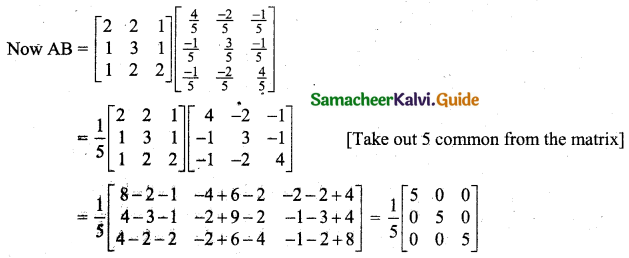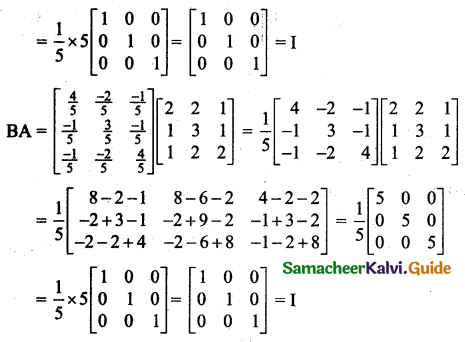Thus AB = BA = I
Hence A and B are inverses of each other.Question 9.
If A = $$\left[\begin{array}{ll} 3 & 7 \\ 2 & 5 \end{array}\right]$$ and B = $$\left[\begin{array}{ll} 6 & 8 \\ 7 & 9 \end{array}\right]$$, then verify that (AB)-1 = B-1A-1
Solution: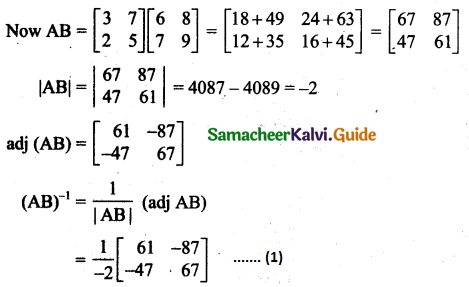Now we will find B-1A-1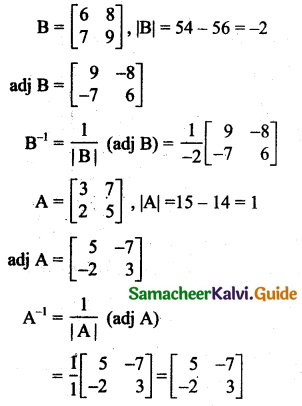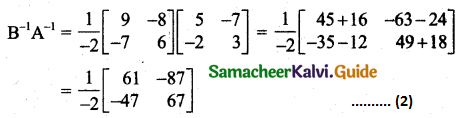From (1) and (2), (AB)-1 = B-1A-1

Question 10.
Find λ if the matrix $$\left[\begin{array}{rrr} 1 & 1 & 3 \\ 2 & \lambda & 4 \\ 9 & 7 & 11 \end{array}\right]$$ has no inverse.
Solution: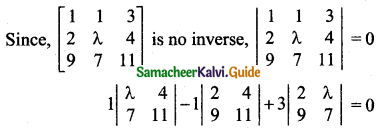1[11λ – 28] – 1[22 – 36] + 3[14 – 9λ] = 0
11λ – 28 + 14 + 42 – 27λ = 0
-16λ + 28 = 0
-16λ = -28
λ = $$\frac{-28}{-16}=\frac{7}{4}$$Question 11.
If X = $$\left[\begin{array}{rrr} 8 & -1 & -3 \\ -5 & 1 & 2 \\ 10 & -1 & -4 \end{array}\right]$$ and Y = $$\left[\begin{array}{rrr} 2 & 1 & -1 \\ 0 & 2 & 1 \\ 5 & p & q \end{array}\right]$$ then, find p, q if Y = X-1
Solution:
Given that Y is the inverse of X.
∴ XY = I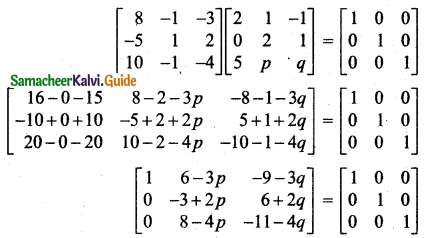6 – 3p = 0 and -9 – 3q = 0
6 = 3p and -9 = 3q
∴ p = 2; q = -3